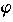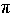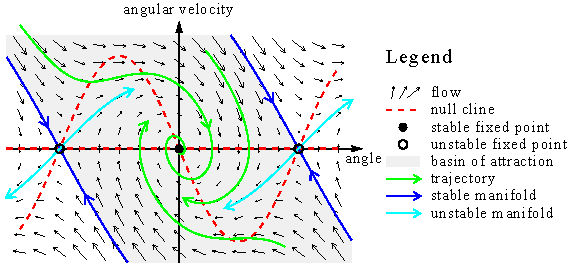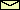# Basic Terms of Nonlinear Dynamics

Nonlinear dynamics is a language to talk about dynamical systems. Here, brief definitions are given for the basic terms of this language. All these terms will be illustrated at the pendulum.

Dynamical system:
A part of the world which can be seen as a self-contained entity with some temporal behavior. In nonlinear dynamics, speaking about a dynamical system usually means to speak about an abstract mathematical system which is a model for such an entity. Mathematically, a dynamical system is defined by its state and by its dynamics. A pendulum is an example for a dynamical system.
State:
A number or a vector (i.e., a list of numbers) defining the state of the dynamical system uniquely. For the undriven pendulum, the state is uniquely defined by the angleand the angular velocity:= d/dt.. In the case of driving, the driving phaseis also needed because the pendulum becomes a nonautonomous system. In spatially extended systems, the state is often a field (a scalar field or a vector field). Mathematically spoken, fields are functions with space coordinates as independent variables. The velocity field of a fluid is a well-known example.
Phase space:
All possible states of the system. Each point in the phase space corresponds to a unique state. In the case of the undriven pendulum, the phase space has two dimensions whereas for driven pendula it has three. The dimension of the phase space is infinite in cases where the system state is defined by a field.
Dynamics or equation of motion:
The causal relation between the present state and the next state in the future. It is a deterministic rule which tells us what happens in the next time step. In the case of a continuous time, the time step is infinitesimally small. Thus, the equation of motion is a differential equation or a system of differential equations:
 du/dt = F(u),
where u is the state and t is the time variable. An example is the equation of motion of an undriven and undamped pendulum. In the case of a discrete time, the time steps are nonzero and the dynamics is a map:
 un+1 = F(un),
with the discrete time n. An example is the baker map. Note, that the corresponding physical time points tn do not necessarily occur equidistantly. Only the order has to be the same. That is, n < m implies tn < tm.
The dynamics is linear if the causal relation between the present state and the next state is linear. Otherwise it is nonlinear.
What happens if the next state isn't uniquely defined by the present one? In general, this is an indication that the phase space is not complete. Thus, there are important variables determining the state which had been forgotten. Of course, this is a crucial point while modelling a real-life systems. But beside this, there are two important classes of systems where the phase space is incomplete: The nonautonomuous systems and the stochastic systems. A nonautonomous systems has an equation of motion which depends explicitly on time. Thus, the dynamical rule governing the next state not only depends on the present state but also at the time it applies. A driven pendulum is an example of a nonautonomuous system. Fortunately, there is an easy way to make the phase space complete. Simply include the time into the definition of the state! Mathematically, this is done by introducing a new state variable. Its dynamics reads d/dt = 1, orn+1 =n, depending on whether time is continuous or discrete. For the periodically driven pendula, it is also natural to take the driving phaseas the new state variable. Its equation of motion reads
 d/dt = 2f,
where f is the driving frequency (the angular driving frequency is 2f).
In a stochastic system, the number and the nature of the variables necessary to complete the phase space is usually unknown. Therefore, the next state can not be deduced from the present one. The deterministic rule is replaced by a stochastic one. Instead of the next state, it gives only the probabilities of all points in the phase space to be the next state.
Orbit or trajectory:
A solution of the equation of motion. In the case of continuous time, it is a curve in phase space parametrized by the time variable. For a discrete system it is an ordered set of points in the phase space.
Flow:
The mapping of the whole phase space of a continuous dynamical system onto itself for a given time step t. If t is an infinitesimal time step dt, the flow is just given by the right-hand side of the equation of motion (i.e., F). In general, the flow for a finite time step is not known analytically because this would be equivalent to have a solution of the equation of motion.As an example, the figure shows the flow of a damped pendulum. The black arrows of the vector field F are tangential at the trajectories. In a two-dimensional phase space, you can draw a qualitative picture of the flow and the orbits. First, draw the so-called null clines. These are the lines were the time derivative of one component of the state variable is zero. Here, one null cline is the angle axes because the time derivative of the angle is zero when the angular velocity is zero. The other null cline is= -02sin/. On these null clines, draw the vector field vertical or horizontal, respectively. Between the null clines draw the vector field in the direction north east, south east, south west, or north west. The direction is determined by the signs of d/dt and d/dt. At the crossing points of the null clines, the vector field is zero, i.e., d/dt = 0 and d/dt = 0. These points are called fixed points. They correspond to stationary solutions. Fixed points are examples of non-wandering sets. They can be either stable or unstable.
Poincaré section and Poincaré map:A carefully chosen (curved) plane in the phase space that is crossed by almost all orbits. It is a tool developed by Henri Poincaré (1854-1912) for a visualization of the flow in a phase space of more than two dimensions. The Poincaré section has one dimension less than the phase space. The Poincaré map maps the points of the Poincaré section onto itself. It relates two consecutive intersection points. Note, that only those intersection points counts which come from the same side of the plane. A Poincaré map turns a continuous dynamical system into a discrete one. If the Poincaré section is carefully chosen no information is lost concerning the qualitative behavior of the dynamics. Poincaré maps are invertable maps because one gets un from un+1 by following the orbit backwards. In the Pendulum Lab, you can turn the oscilloscope into a special Poincaré map called stroboscopic map were the Poincaré section is defined by a certain phase of the time-periodic driving.
Non-wandering set:
A set of points in the phase space having the following property: All orbits starting from a point of this set come arbitrarily close and arbitrarily often to any point of the set. Non-wandering sets are coming in four flavors:
1. Fixed points:Stationary solutions. For the undriven pendulum and for the vertically driven pendulum,= 0, d/dt = 0 and= 180°, d/dt = 0 are fixed points.
2. Limit cycles:Periodic solutions. These solutions are common in weakly driven pendula.
3. Quasiperiodic orbits: Periodic solutions with at least two incommensurable frequencies (i.e., the ratio of the frequencies is an irrational number). In the Pendulum Lab, these solutions occur only in undamped but driven pendula.
4. Chaotic orbits: Bound non-periodic solutions. These solutions occur in driven pendula if the driving is strong enough.
The first three types can also occur in linear dynamics. The fourth type appears only in nonlinear systems. Its possibility was first anticipated by the genius of Henri Poincaré (1854-1912). In the meanwhile, computers had turned this previously counterintuitive behavior into a widespread experience. In the seventies, this irregular behavior was termed deterministic chaos. In the Poincaré map, limit cycles become fixed points. A non-wandering set can be either stable or unstable. Changing a parameter of the system can change the stability of a non-wandering set. This is accompanied by a change of the number of non-wandering sets due to a bifurcation.

© 1998 Franz-Josef Elmer,Franz-Josef doht Elmer aht unibas doht ch, last modified Wednesday, July 22, 1998.